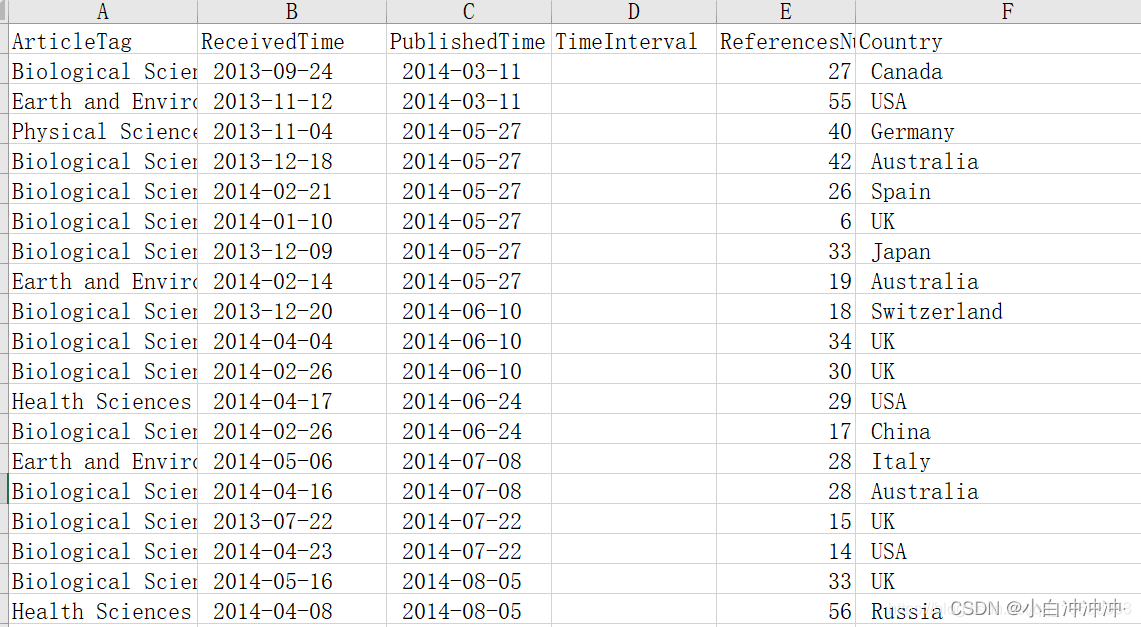# pandas apply() 函数用法

### lambda

``````def can_divide_by_three(number):
if number % 3 == 0:
return True
else:
return False

selected_numbers = []
for number in range(1, 11):
if can_divide_by_three(number):
selected_numbers.append(number)
``````

``````divide_by_three = lambda x : True if x % 3 == 0 else False

selected_numbers = []
for number in range(1, 11):
if divide_by_three(item):
selected_numbers.append(item)
``````

Python 语言提供 filter() 函数，语法如下：

``````filter(function, sequence)
``````

filter() 函数功能：对 sequence 中的 item 依次执行 function(item)，将结果为 True 的 item 组成一个 List/String/Tuple（取决于 sequence 的类型）并返回。有了这个函数，上面的代码可以简化为：

``````divide_by_three = lambda x : True if x % 3 == 0 else False
selected_numbers = filter(divide_by_three, range(1, 11))

#将 lambda 表达式放在语句中，代码简化到只需要一句话就够了
selected_numbers = filter(lambda x: x % 3 == 0, range(1, 11))
``````

### Series.apply()

``````  Name Nationality  score
张           汉    400
李           回    450
王           汉    460
``````

``````import pandas as pd

df['Extrascore'] = df['Nationality'].apply(lambda x : 5 if x != '汉' else 0)
df['Totalscore'] = df['score'] + df['Extrascore']
``````

``````  Name Nationality  score  Extrascore  Totalscore
0    张           汉    400           0         400
1    李           回    450           5         455
2    王           汉    460           0         460
``````

apply() 函数当然也可执行 python 内置的函数，比如我们想得到 Name 这一列字符的个数，如果用 apply() 的话：

``````df['NameLength'] = df['Name'].apply(len)
``````

### DataFrame.apply()

apply函数`pandas`里面所有函数中自由度最高的函数。该函数如下：

args=(), **kwds)

DataFrame.apply() 函数则会遍历每一个元素，对元素运行指定的 function。比如下面的示例：

``````import pandas as pd
import numpy as np

matrix = [
[1,2,3],
[4,5,6],
[7,8,9]
]

df = pd.DataFrame(matrix, columns=list('xyz'), index=list('abc'))
df.apply(np.square)
``````

``````    x   y   z
a   1   4   9
b  16  25  36
c  49  64  81
``````

``````df.apply(lambda x : np.square(x) if x.name=='x' else x)

x  y  z
a   1  2  3
b  16  5  6
c  49  8  9
``````

``````df.apply(lambda x : np.square(x) if x.name in ['x', 'y'] else x)

x   y  z
a   1   4  3
b  16  25  6
c  49  64  9
``````

``````df.apply(lambda x : np.square(x) if x.name == 'a' else x, axis=1)
``````

### apply() 计算日期相减示例

``````    wbs   date_from     date_to
job1  2019-04-01  2019-05-01
job2  2019-04-07  2019-05-17
job3  2019-05-16  2019-05-31
job4  2019-05-20  2019-06-11
``````

``````import pandas as pd
import datetime as dt

wbs = {
"wbs": ["job1", "job2", "job3", "job4"],
"date_from": ["2019-04-01", "2019-04-07", "2019-05-16","2019-05-20"],
"date_to": ["2019-05-01", "2019-05-17", "2019-05-31", "2019-06-11"]
}

df = pd.DataFrame(wbs)
df['elpased'] = df['date_to'].apply(pd.to_datetime) -
df['date_from'].apply(pd.to_datetime)
``````

apply() 函数将 date_from 和 date_to 两列转换成 datetime 类型。我们 print 一下 df:

``````    wbs   date_from     date_to elapsed
0  job1  2019-04-01  2019-05-01 30 days
1  job2  2019-04-07  2019-05-17 40 days
2  job3  2019-05-16  2019-05-31 15 days
3  job4  2019-05-20  2019-06-11 22 days
``````

``````df['elpased']= df['date_to'].apply(pd.to_datetime) -
df['date_from'].apply(pd.to_datetime)
df['elapsed'] = df['elpased'].apply(lambda x : x.days)
``````

``````import pandas as pd
import datetime as dt

def get_interval_days(arrLike, start, end):
start_date = dt.datetime.strptime(arrLike[start], '%Y-%m-%d')
end_date = dt.datetime.strptime(arrLike[end], '%Y-%m-%d')

return (end_date - start_date).days

wbs = {
"wbs": ["job1", "job2", "job3", "job4"],
"date_from": ["2019-04-01", "2019-04-07", "2019-05-16","2019-05-20"],
"date_to": ["2019-05-01", "2019-05-17", "2019-05-31", "2019-06-11"]
}

df = pd.DataFrame(wbs)
df['elapsed'] = df.apply(
get_interval_days, axis=1, args=('date_from', 'date_to'))
``````

### apply例子``````import pandas as pd
import datetime   #用来计算日期差的包

def dataInterval(data1,data2):
d1 = datetime.datetime.strptime(data1, '%Y-%m-%d')
d2 = datetime.datetime.strptime(data2, '%Y-%m-%d')
delta = d1 - d2
return delta.days

def getInterval(arrLike):  #用来计算日期间隔天数的调用的函数
PublishedTime = arrLike['PublishedTime']
return days

if __name__ == '__main__':
fileName = "NS_new.xls";
df['TimeInterval'] = df.apply(getInterval , axis = 1)

``````

``````import pandas as pd
import datetime   #用来计算日期差的包

def dataInterval(data1,data2):
d1 = datetime.datetime.strptime(data1, '%Y-%m-%d')
d2 = datetime.datetime.strptime(data2, '%Y-%m-%d')
delta = d1 - d2
return delta.days

def getInterval_new(arrLike,before,after):  #用来计算日期间隔天数的调用的函数
before = arrLike[before]
after = arrLike[after]
days = dataInterval(after.strip(),before.strip())  #注意去掉两端空白
return days

if __name__ == '__main__':
fileName = "NS_new.xls";
df['TimeInterval'] = df.apply(getInterval_new ,
axis = 1, args = ('ReceivedTime','PublishedTime'))    #调用方式一
#下面的调用方式等价于上面的调用方式
df['TimeInterval'] = df.apply(getInterval_new ,
#下面的调用方式等价于上面的调用方式
df['TimeInterval'] = df.apply(getInterval_new ,

``````

### 相关推荐

IT行业是什么工作做什么？IT行业的工作有：产品策划类、页面设计类、前端与移动、开发与测试、营销推广类、数据运营类、运营维护类、游戏相关类等，根据不同的分类下面有细分了不同的岗位。